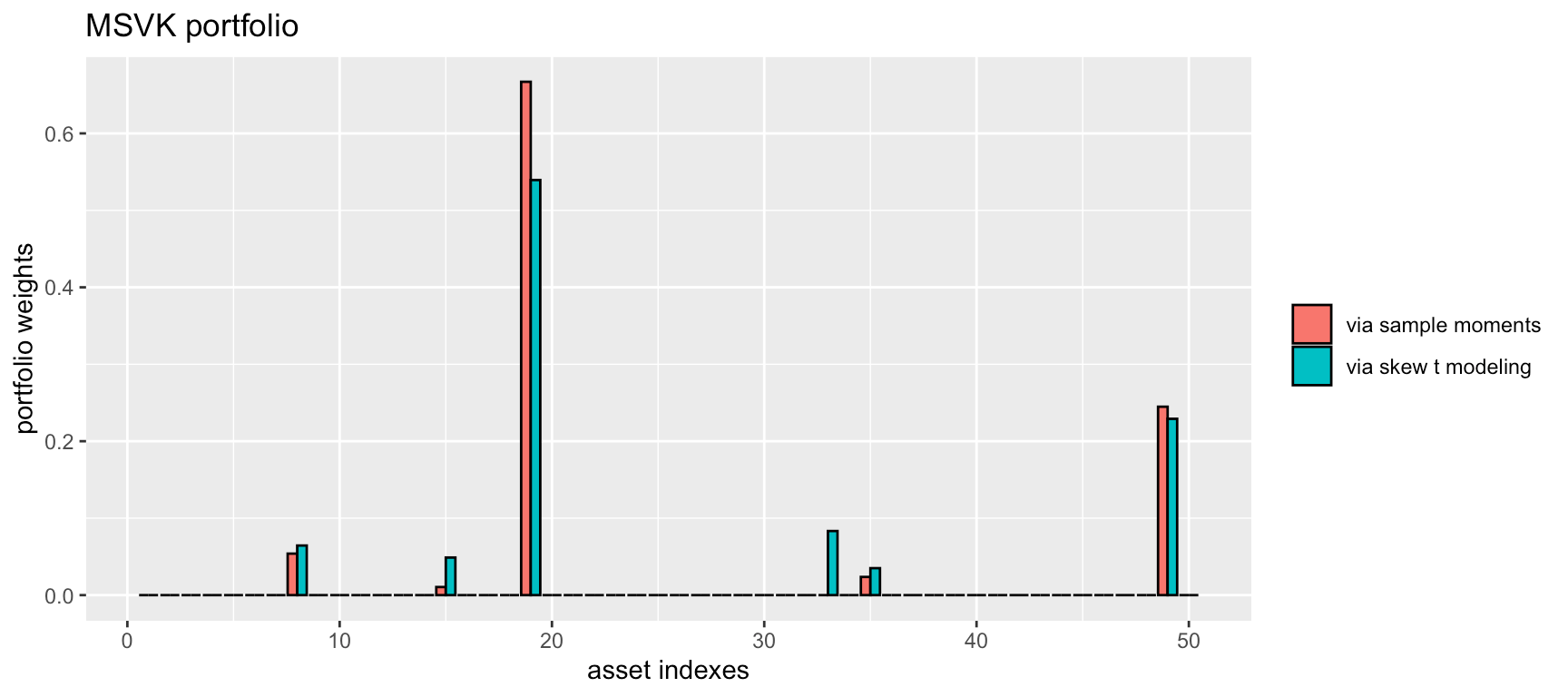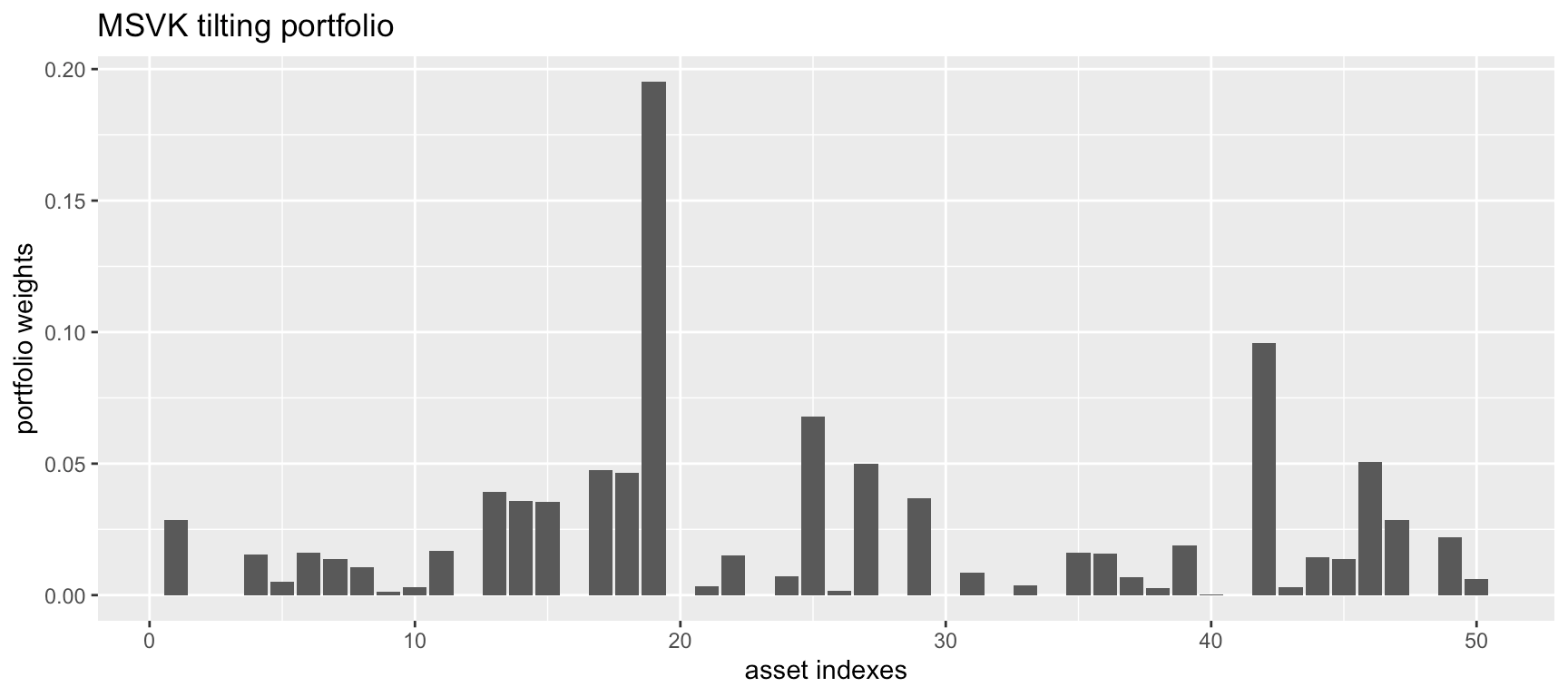This vignette illustrates the design of high order portfolios with the package highOrderPortfolios.

# Quick Start

library(highOrderPortfolios)

# get help for the functions
?design_MVSK_portfolio_via_sample_moments
#> ℹ Rendering development documentation for "design_MVSK_portfolio_via_sample_moments"
?design_MVSK_portfolio_via_skew_t
#> ℹ Rendering development documentation for "design_MVSK_portfolio_via_skew_t"

The simplest use is for the mean-variance-skewness-kurtosis (MVSK) portfolio (using the provided dataset X50):

# non-parametric case: estimate sample moments
X_moments <- estimate_sample_moments(X50)

# parametric case: estimate the multivariate skew t distribution
X_skew_t_params <- estimate_skew_t(X50)

# choose hyper-parameter moment weights for the MVSK formulation
xi <- 10
lmd <- c(1, xi/2, xi*(xi+1)/6, xi*(xi+1)*(xi+2)/24)

# design portfolio
sol_nonparam <- design_MVSK_portfolio_via_sample_moments(lmd, X_moments)
sol_param    <- design_MVSK_portfolio_via_skew_t(lmd, X_skew_t_params)

We can now plot the designed MVSK portfolios (both the non-parametric and parametric cases):

library(ggplot2)

rbind(data.frame(idx = 1:ncol(X50), weights = sol_nonparam$w, type = "via sample moments"), data.frame(idx = 1:ncol(X50), weights = sol_param$w,    type = "via skew t modeling")) |>
ggplot(aes(x = idx, y = weights, fill = type)) +
geom_bar(color = "black", stat = "identity", position = "dodge", width = 0.9) +
labs(title = "MSVK portfolio", x = "asset indexes", y = "portfolio weights") +
theme(legend.title = element_blank())# What is a High-Order Portfolio?

## Signal model

Let us denote by $$\boldsymbol{r}_{t}$$ the vector of returns of $$N$$ assets at time $$t$$ and suppose they follow an i.i.d. distribution (which is not a totally accurate assumption, but it is widely adopted, nonetheless) with mean $$\boldsymbol{\mu}$$ and covariance matrix $$\boldsymbol{\Sigma}$$.

The portfolio vector $$\boldsymbol{w}$$ denotes the normalized dollar weights allocated to the $$N$$ assets, and the portfolio return is then $$r_{t}^\textsf{portf} = \boldsymbol{w}^{\mkern-2mu\raise-1mu\textsf{T}}\boldsymbol{r}_{t}$$, with expected return $$\boldsymbol{w}^{\mkern-2mu\raise-1mu\textsf{T}}\boldsymbol{\mu}$$ and variance $$\boldsymbol{w}^{\mkern-2mu\raise-1mu\textsf{T}}\boldsymbol{\Sigma}\boldsymbol{w}$$.

## Modern portfolio theory

In 1952, Markowitz proposed in his seminal paper to find a trade-off between the portfolio expected return and its risk measured by the variance: $\begin{array}{ll} \underset{\boldsymbol{w}}{\textsf{maximize}} & \boldsymbol{w}^{\mkern-2mu\raise-1mu\textsf{T}}\boldsymbol{\mu} - \lambda\boldsymbol{w}^{\mkern-2mu\raise-1mu\textsf{T}}\boldsymbol{\Sigma}\boldsymbol{w}\\ \textsf{subject to} & \boldsymbol{w}\in\mathcal{W}, \end{array}$ where $$\lambda$$ is a hyper-parameter that controls how risk-averse the investor is and $$\mathcal{W}$$ denotes the feasible set such as $$\mathcal{W} \triangleq \{\boldsymbol{w} \mid \boldsymbol{w} \ge \boldsymbol{0}, \boldsymbol{1}^{\mkern-2mu\raise-1mu\textsf{T}}\boldsymbol{w}=1\}$$.

Markowitz’s portfolio has been heavily criticized for over half a century and has never been fully embraced by practitioners, among many other reasons because:

• the mean and variance of the portfolio returns may be acceptable measures when the returns are Gaussian distributed; but the empirical distribution of the portfolio returns is usually asymmetric and heavy-tailed, which cannot be well described by only the mean and variance; and

• the objective $$\boldsymbol{w}^{\mkern-2mu\raise-1mu\textsf{T}}\boldsymbol{\mu} - \lambda\boldsymbol{w}^{\mkern-2mu\raise-1mu\textsf{T}}\boldsymbol{\Sigma}\boldsymbol{w}$$ assumes that investors have a taste for the quadratic expected utility function on portfolio return, but investors’ tastes might be very different.

## From mean-variance portfolio to high-order portfolio

To make up for the two aforementioned drawbacks of the mean-variance framework, we need to take high-order moments of the portfolio return into consideration, which allows to take into account:

• the asymmetry and heavy-tailedness of portfolio returns, captured by the third and fourth central moments, respectively, i.e., skewness and kurtosis; and

• approximation of general expected utility functions via the Taylor series expansion truncated to the four most important order terms (i.e., based on the high-order moments).

Generally speaking, a positive skewness usually means the right tail is more heavier than the left tail, while the lower kurtosis usually corresponds to thinner tails at both sides. We can extend the mean-variance framework by directly incorporating the high-order moments to obtain the mean-variance-skewness-kurtosis framework, where we shall try to strike a balance between maximizing the mean and skewness (odd moments) while minimizing the variance and kurtosis (even moments).

## High-order portfolios

The package highOrderPortfolios implements the algorithms designed in the papers and . The two problem formulations covered are the mean-variance-skewness-kurtosis (MVSK) portfolio and the MVSK tilting portfolio.

### MVSK portfolio

The simplest formulation for the high-order portfolio is directly extending the Markowitz portfolio objective by considering also the skewness and kurtosis: $\begin{array}{ll} \underset{\boldsymbol{w}}{\textsf{minimize}} & -\lambda_{1}\phi_{1}(\boldsymbol{w}) + \lambda_{2}\phi_{2}(\boldsymbol{w}) - \lambda_{3}\phi_{3}(\boldsymbol{w}) + \lambda_{4}\phi_{4}(\boldsymbol{w})\\ \textsf{subject to} & \boldsymbol{w}\in\mathcal{W}, \end{array}$ where $$\lambda_{1},\lambda_{2},\lambda_{3},\lambda_{4}\ge0$$ are the hyper-parameters for combining the four moments of the portfolio returns \begin{aligned} \phi_{1}(\boldsymbol{w}) &= \boldsymbol{w}^{\mkern-2mu\raise-1mu\textsf{T}}\boldsymbol{\mu}\\ \phi_{2}(\boldsymbol{w}) &= \boldsymbol{w}^{\mkern-2mu\raise-1mu\textsf{T}}\boldsymbol{\Sigma}\boldsymbol{w}\\ \phi_{3}(\boldsymbol{w}) &= \boldsymbol{w}^{\mkern-2mu\raise-1mu\textsf{T}}\boldsymbol{\Phi}(\boldsymbol{w}\otimes\boldsymbol{w})\\ \phi_{4}(\boldsymbol{w}) &= \boldsymbol{w}^{\mkern-2mu\raise-1mu\textsf{T}}\boldsymbol{\Psi}(\boldsymbol{w}\otimes\boldsymbol{w}\otimes\boldsymbol{w}), \end{aligned} where $$\boldsymbol{\Phi} \in \mathbb{R}^{N \times N^2}$$ is the co-skewness matrix, and $$\boldsymbol{\Psi} \in \mathbb{R}^{N \times N^3}$$ is the co-kurtosis matrix.

### MVSK tilting portfolio

Another formulation for the high-order portfolio is obtained by moving the high-order moments in the objective down to the constraints; for example, the MVSK tilting portfolio : $\begin{array}{ll} \underset{\boldsymbol{w},\delta}{\textsf{maximize}} & \delta\\ \textsf{subject to} & \phi_{1}(\boldsymbol{w})\ge\phi_{1}(\boldsymbol{w}_{0})+d_{1}\delta,\\ & \phi_{2}(\boldsymbol{w})\le\phi_{2}(\boldsymbol{w}_{0})-d_{2}\delta,\\ & \phi_{3}(\boldsymbol{w})\ge\phi_{3}(\boldsymbol{w}_{0})+d_{3}\delta,\\ & \phi_{4}(\boldsymbol{w})\le\phi_{4}(\boldsymbol{w}_{0})-d_{4}\delta,\\ & (\boldsymbol{w} - \boldsymbol{w}_{0})^{\mkern-2mu\raise-1mu\textsf{T}}\boldsymbol{\Sigma}(\boldsymbol{w} - \boldsymbol{w}_{0})\le\kappa^{2},\\ & \boldsymbol{w}\in\mathcal{W},\delta\ge0, \end{array}$ where $$\boldsymbol{d}=\left[d_{1},d_{2},d_{3},d_{4}\right]\ge\boldsymbol{0}$$ is the tilting direction and $$\kappa^{2}$$ determines the maximum tracking error volatility of $$\boldsymbol{w}$$ with respect to the reference portfolio $$\boldsymbol{w}_{0}$$.

# Using the Package highOrderPortfolios

## Estimate the high-order sample moments

Unlike the mean and covariance matrix, the co-skewness and co-kurtosis matrix are rarely estimated. The base R does not provide an embedded function for estimating them. Therefore, we include the function estimate_sample_moments() in this package (which in turn relies on the package PerformanceAnalytics) to help estimate the sample co-skewness and co-kurtosis matrices:

library(highOrderPortfolios)

# non-parametric case: estimate sample moments
X_moments <- estimate_sample_moments(X50)
names(X_moments)
#>  "mu"        "Sgm"       "Phi_mat"   "Psi_mat"   "Phi"       "Psi"       "Phi_shred" "Psi_shred"

## Fit a multivariate skew $$t$$ distribution

As a more time-efficient alternative, we can use a parametric approach by fitting the data matrix to a multivariate skew $$t$$ distribution with the function estimate_skew_t() (which in turn relies on the package fitHeavyTail):

# parametric case: estimate the multivariate skew t distribution
X_skew_t_params <- estimate_skew_t(X50)
names(X_skew_t_params)
#>  "mu"         "nu"         "gamma"      "scatter"    "chol_Sigma" "a"

## MVSK portfolio

To design the MVSK portfolio, first we need to choose the value of the hyper-parameters that weight each of the four moments:

# choose hyper-parameter moment weights for the MVSK formulation
xi <- 10
lmd <- c(1, xi/2, xi*(xi+1)/6, xi*(xi+1)*(xi+2)/24)

Then we can design the MVSK portfolio via the non-parametric sample moments:

sol_nonparam <- design_MVSK_portfolio_via_sample_moments(lmd, X_moments)

or via the parametric skew $$t$$ modeling:

sol_param    <- design_MVSK_portfolio_via_skew_t(lmd, X_skew_t_params)

We can now plot the designed MVSK portfolios (both the non-parametric and parametric cases):

library(ggplot2)

rbind(data.frame(idx = 1:ncol(X50), weights = sol_nonparam$w, type = "via sample moments"), data.frame(idx = 1:ncol(X50), weights = sol_param$w,    type = "via skew t modeling")) |>
ggplot(aes(x = idx, y = weights, fill = type)) +
geom_bar(color = "black", stat = "identity", position = "dodge", width = 0.9) +
labs(title = "MSVK portfolio", x = "asset indexes", y = "portfolio weights") +
theme(legend.title = element_blank())## MVSK tilting portfolio

Similarly, the MVSK tilting portfolio can be designed based on the non-parametric sample moments:

# estimate sample moments
X_moments <- estimate_sample_moments(X50, adjust_magnitude = TRUE)

# choose hyper-parameters in the problem setting
w0 <- rep(1/50, 50)
w0_moments <- eval_portfolio_moments(w0, X_moments)
d <- abs(w0_moments)
kappa <- 0.3 * sqrt(w0 %*% X_moments$Sgm %*% w0) # portfolio design sol_nonparam <- design_MVSKtilting_portfolio_via_sample_moments(d, X_moments, w_init = w0, w0 = w0, w0_moments = w0_moments, kappa = kappa) We can now plot the designed MVSK tilting portfolio: rbind(data.frame(idx = 1:ncol(X50), weights = sol_nonparam$w)) |>
ggplot(aes(x = idx, y = weights)) +
geom_bar(stat = "identity", position = "dodge", width = 0.9) +
labs(title = "MSVK tilting portfolio", x = "asset indexes", y = "portfolio weights") +
theme(legend.title = element_blank())